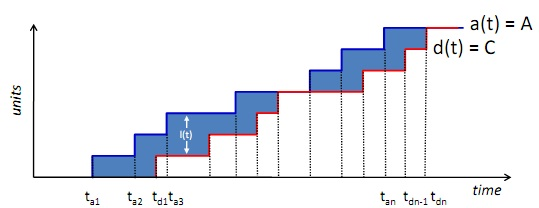Assumptions in Queuing system

Assumptions in Queuing system:

Flow balance implies that the number of arrivals in an observation period is equal to the number of completions in the same period (λ = X)

One step behavior asserts that no two jobs in the system "change state" (i.e., finish processing at some device or arrive to the system) at exactly the same time

Homogeneity means that the average job-arrival rate and the average service rate are independent of the system’s state. Furthermore the probability of moving between two servers is independent of the numbers of jobs at the source and destination servers. Unlike the other two assumptions, violating the homogeneity assumptions  introduces considerable error in the calculations.

#### Related Questions in Basic Statistics

• ##### Q :Define Service Demand Law

Service Demand Law:

• Dk = SKVK, Average time spent by a typical request obtaining service from resource k

• DK = (ρk/X

• ##### Q :State Kendalls notation

Kendall’s notation:  A/B/C/K/m/Z

A, Inter-arrival distribution

• M exponential
• D constant or determ

• ##### Q :Assumptions in Queuing system

Assumptions in Queuing system:

Flow balance implies that the number of arrivals in an observation period is equal to the

• ##### Q :FIN512 Entrepreneurial Finance Chapter

Chapter 6: Discussion Question: #4 p. 223

It is usually easier to forecast sales for a seasoned firm contrast to an early-stage venture because an early-stage venture has limited access to bank credit lines, sho

##### Q :Cumulative Frequency and Relative

Explain differences between Cumulative Frequency and Relative Frequency?

• ##### Q :State Littles Law Little’s Law : • L =

Little’s Law:

• L = λR = XR

• Lq = λW = XW

• Little’s Law holds as long as customers are not destroyed or&nbs

• ##### Q :Computing Average revenue using

Can anyone help me in the illustrated problem? The airport branch of a car rental company maintains a fleet of 50 SUVs. The inter-arrival time between the requests for an SUV is 2.4 hrs, on an average, with a standard deviation of 2.4 hrs. There is no indication of a

• ##### Q :Simplified demonstration of Littles Law

Simplified demonstration of Little’s Law:##### Q :Develop the most appropriate regression

Predicting Courier Costs

The law firm of Adams, Babcock, and Connors is located in the Dallas-Fort metroplex.  Randall Adams is the senior and founding partner of the firm.  John Babcock has been a partne